# 数据抓取实践：对加密参数及压缩混淆JS的逆向分析

## 1. 一夫当关 – XHR Breakpoints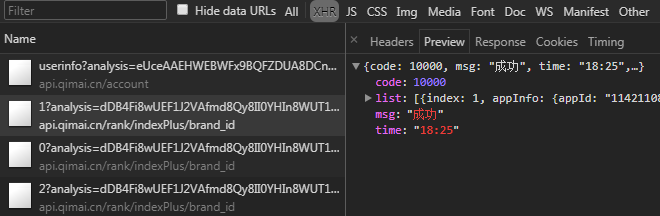```analysis: dDB4Fi8wUEF...(太长，略)
brand: all
country: cn
device: iphone
genre: 36
date: 2018-05-14
page: 1
```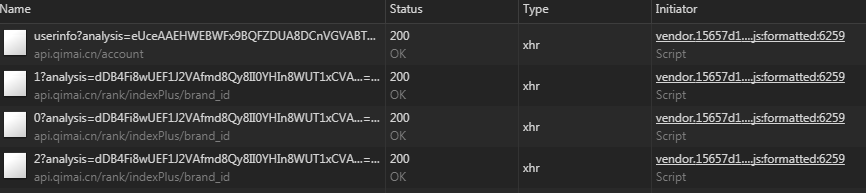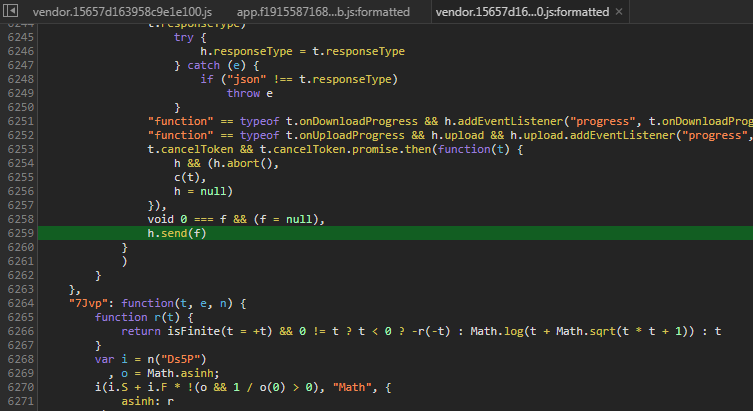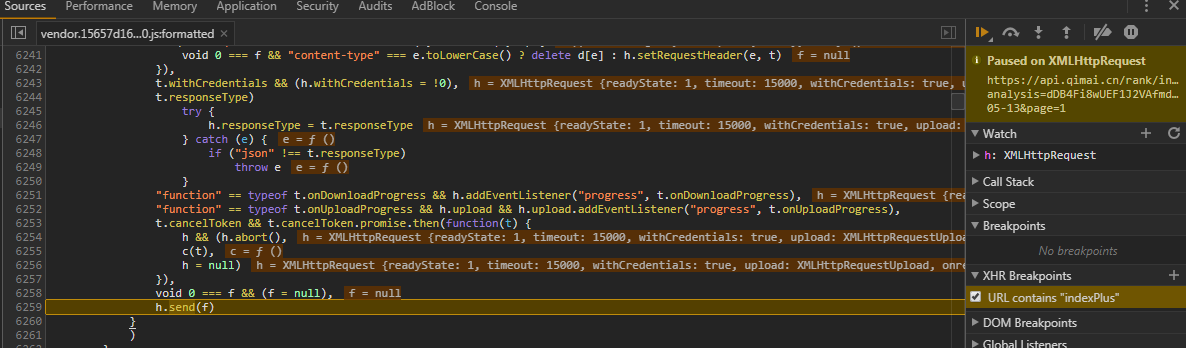## 2. 穿针引线 – Module Require

```"7O1s": function(t, e, n) {
var r = n("DIVP")
, i = n("XSOZ")
, o = n("kkCw")("species");
t.exports = function(t, e) {
var n, a = r(t).constructor;
return void 0 === a || void 0 == (n = r(a)[o]) ? e : i(n)
}
},
"7UMu": function(t, e, n) {
var r = n("R9M2");
t.exports = Array.isArray || function(t) {
return "Array" == r(t)
}
},
"7gX0": function(t, e) {
var n = t.exports = {
version: "2.5.5"
};
"number" == typeof __e && (__e = n)
},
```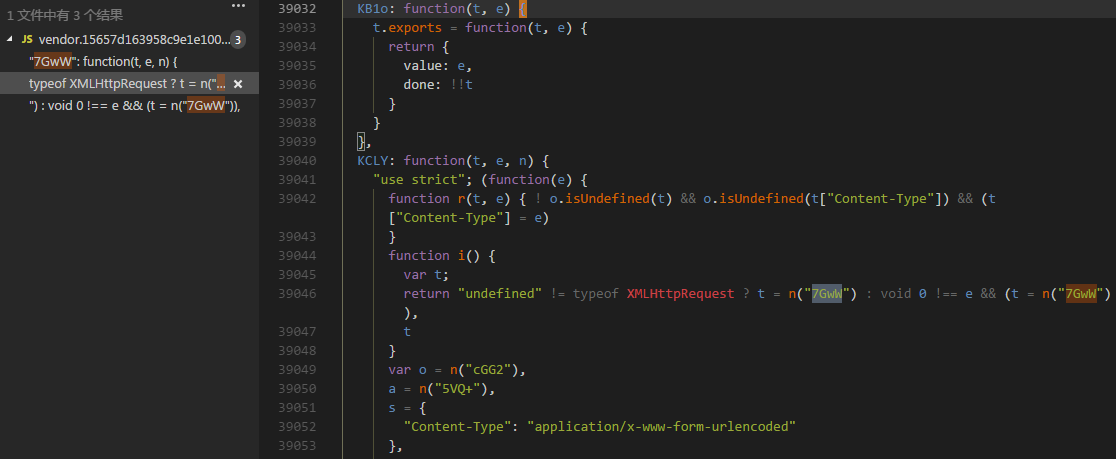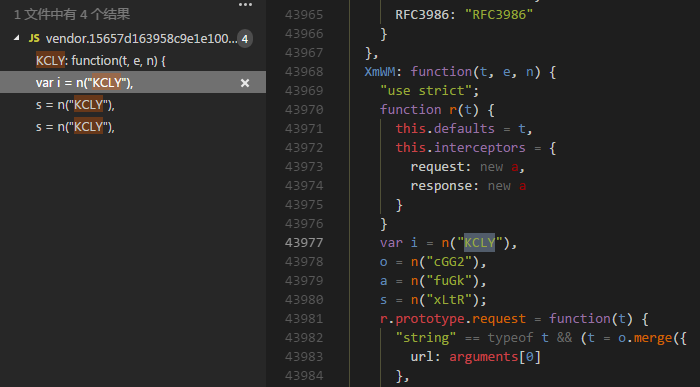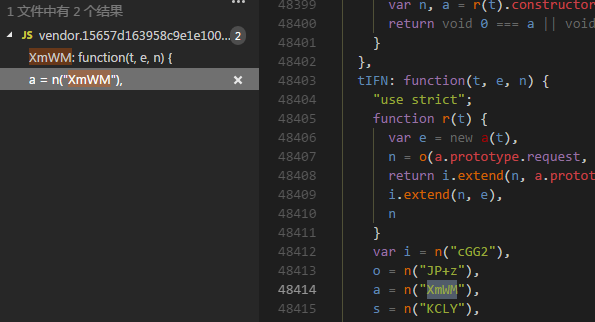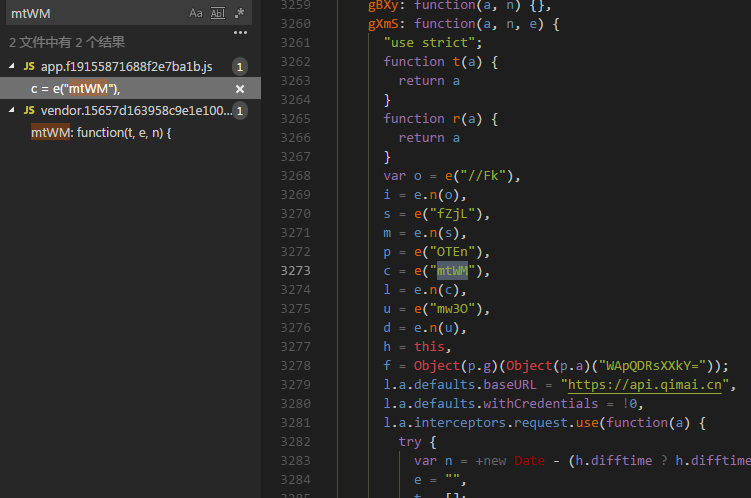## 3. 顺藤摸瓜 – Call Stack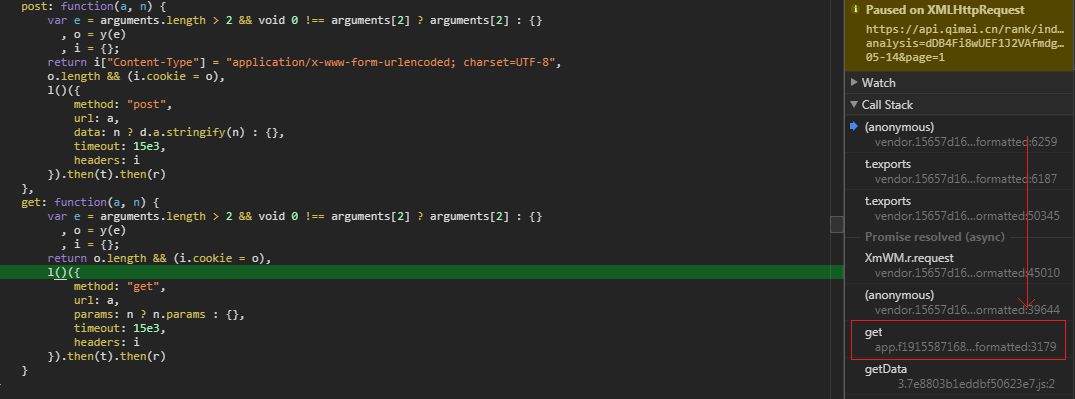get 方法即是模块 `gXmS` 中的函数。需要注意的是，此方法在模块的末尾，如果不细心可能会错过。

## 4. 偷天换日 – Hijack The Response```l.a.interceptors.request.use(function(a) {
try {
var n = +new Date - (h.difftime ? h.difftime : 0) - 1515125653845
, e = ""
, t = [];
return void 0 === a.params && (a.params = {}),
m()(a.params).forEach(function(n) {
if (n == f)
return !1;
a.params.hasOwnProperty(n) && t.push(a.params[n])
}),
t = t.sort().join(""),
t = Object(p.d)(t),
t += "@#" + a.url.replace(a.baseURL, ""),
t += "@#" + n,
t += "@#1",
e = Object(p.d)(Object(p.g)(t)),
-1 == a.url.indexOf(f) && (a.url += "?" + f + "=" + encodeURIComponent(e)),
a
} catch (a) {}
}, function(a) {
return i.a.reject(a)
}),
```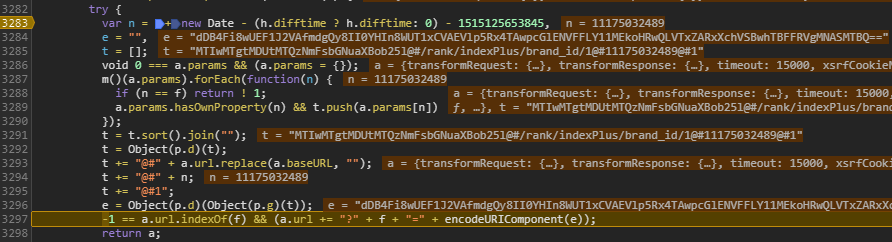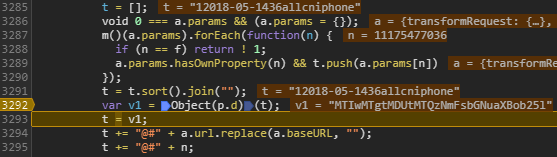1. 设置一个时间差变量
2. 提取查询参数值（除了 analysis）
3. 排序拼接参数值字符串并 Base64 编码
4. 拼接自定义字符串
5. 自定义加密后再 Base64 编码
6. 拼接 URL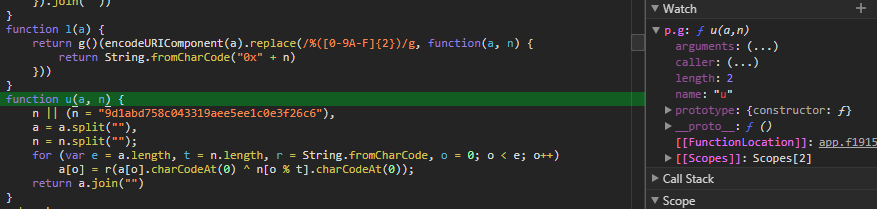## 5. 东搜西罗 – Searching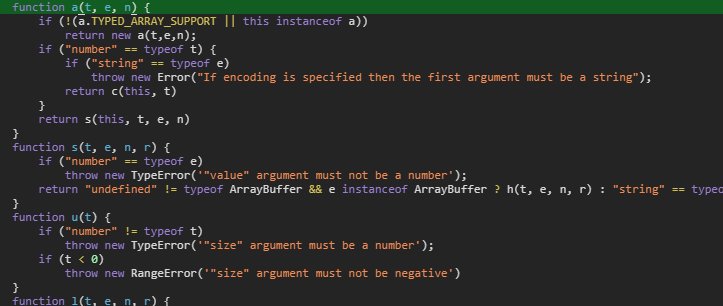## 6. 一锤定音 – Crawler

```#!/usr/bin/env python3

import time
import json
import base64
import requests
from urllib.parse import urlencode

"Accept": "application/json, text/plain, */*",
"Referer": "https://www.qimai.cn/rank",
"User-Agent": "Mozilla/5.0 (Windows NT 6.1; Win64; x64; rv:57.0) Gecko/20100101 Firefox/59.0"
}

params = {
"brand": "all",
"country": "cn",
"date": "2018-05-14",
"device": "iphone",
"genre": "36",
"page": 1
}

# 自定义加密函数
def encrypt(a, n="9d1abd758c043319aee5ee1c0e3f26c6"):
s = list(a)
n = list(n)
sl = len(s)
nl = len(n)
for i in range(0, sl):
s[i] = chr(ord(s[i]) ^ ord(n[i % nl]))
return "".join(s)

def main():
# iPhone 免费榜单
# 提取查询参数值并排序
s = "".join(sorted([str(v) for v in params.values()]))
# Base64 Encode
s = base64.b64encode(bytes(s, encoding="ascii"))
# 时间差
t = str(int((time.time() * 1000 - 1515125653845)))
# 拼接自定义字符串
s = "@#".join([s.decode(), "/rank/indexPlus/brand_id/1", t, "1"])
# 自定义加密 & Base64 Encode
s = base64.b64encode(bytes(encrypt(s), encoding="ascii"))
# 拼接 URL
params["analysis"] = s.decode()
url = "https://api.qimai.cn/rank/indexPlus/brand_id/1?{}".format(urlencode(params))
# 发起请求
print(j)

if __name__ == '__main__':
main()
```

EOF

(0)